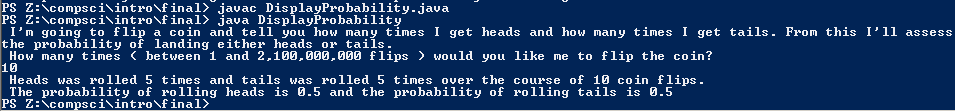# Semester 1 Final

## Code

```      ///Name: Dakota Donahue
///Period: 5
///Project Name: Semester 1 Final
///File Name: DisplayProbability.java
///Date: 1/20/2016

import java.util.Scanner;
import java.util.Random;

public class DisplayProbability
{
public static void main( String[] args )
{
Scanner keyboard = new Scanner(System.in);

Random r = new Random();

System.out.println( " I'm going to flip a coin and tell you how many times I get heads and how many times I get tails. From this I'll assess the probability of landing either heads or tails. " );

System.out.println( " How many times ( between 1 and 2,100,000,000 flips ) would you like me to flip the coin? " );
int flips = keyboard.nextInt();

while ( flips < 1 || flips > 2100000000 )
{
if ( flips < 1 )
{
System.out.println ( " This isn't a sufficient number of coin flips " );
}
else
{
System.out.println ( " You've asked me to do too many coin flips. " );
}
System.out.println( " How many times ( between 1 and 2,100,000,000 flips ) would you like me to flip the coin? " );
flips = keyboard.nextInt();
}

int result = 0;
int numberOfTails = 0;
int numberOfFlips = 0;

while ( numberOfFlips < flips )
{
numberOfFlips++;

result = r.nextInt(2);

numberOfTails = numberOfTails + result;
}

int numberOfHeads = numberOfFlips - numberOfTails;

System.out.println( " Heads was rolled " + numberOfHeads + " times and tails was rolled " + numberOfTails + " times over the course of " + numberOfFlips + " coin flips. " );

double probOfTails = (double)numberOfTails / numberOfFlips;

System.out.println( " The probability of rolling heads is " + probOfHeads + " and the probability of rolling tails is " + probOfTails );
}
}

// In order to keep track of number of flips completed I used two variables for flips completed and flips requested by the user. I consistently referred to the final variables as "numberOf..." for logical continuity.

//I was able to consistently get a 50% heads and 50% tails ratio from 10 coin flips; anything lower than that frequently would result in either a 100%, 0% ratio, a 67%, 33% ration, or a 60%, 40% ratio.

```

### Picture of the output